# SSC CGL EXAMS 2018 | Reasoning Ability Practice Questions (Day-6)

Dear Aspirants, Here we have given the Important SSC CGL Exam 2018 Practice Test Papers. Candidates those who are preparing for SSC CGL 2018 can practice these questions to get more confidence to Crack SSC CGL 2018 Examination.

[WpProQuiz 4129]

1) If 21 + 21 = 4416,

22 + 22 = 4846, then

Find 18 + 18 =?

a) 1824

b) 4448

c) 3246

d) 8181

2) Arrange following as per English dictionary order.

i) Still

ii) Steel

iii) Steal

iv) Stick

a) d, a, c, b

b) c, b, d, a

c) b, c, a, d

d) a, b, c, d

3) Select the missing number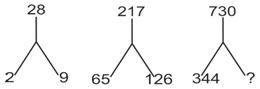a) 513

b) 500

c) 576

d) 396

4) If PAINTING is coded as NIAPGNIT then, CLEARING is codes as

a) GNIRCLEA

b) LCAEGNIR

c) GNIRAELC

d) AELCGNIR

5) Select the related word from the given alternatives.

Elephant: Trumpets :: Donkey : ?

a) Chatters

b) Brays

c) Grunts

d) Bellow

6) Ashish is taller than Prakash. Pramod is shorter than Prakash. Amir is shorter than Ashish. Amir is taller than Pramod. Who among the following is shortest?

a) Prakash

b) Amir

c) Pramod

d) Ashish

7) Select the related number from given alternative.

1 : 27 : : ? : 125

a) 64

b) 27

c) 81

d) 25

8) Find the word that cannot be formed from the letters in the given word CONCLUSIONS.

a) COUSINS

b) CLOUDS

c) COUNCIL

d) NOUNS

9) Petter, during the time of sunrise, started walking towards the opposite of sun and then turns right and right again what direction is he facing?

a) South

b) West

c) East

d) North

Directions: (Q. 10-12)

P and Q are brother of R. Q is son of S and T. S is daughter of U. A is the father in law of T. B is son of U.

10)  What is the relationship of Q to B?

a) Nephew

b) Niece

c) Maternal uncle

d) Paternal Aunt

11) What is relationship of P to B.

a) Nephew

b) Paternal uncle

c) Niece

d) Paternal Aunt

12) What is relationship of P to A?

a) Grandson

b) Son

c) Daughter

d) Father

13) Find the missing term in series

2, 6, 24, 120, 720 ,……..

1) 4320

2) 3600

3) 5040

4) 2880

14) How many squares are there in the square figure ABCD?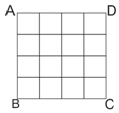a) 18

b) 17

c) 30

d) 26

15) Select the related letters from the given alternatives:-

DHL : PTX : : BFJ :

a) KOS

b) NRV

c) NRU

d) NPS

21 + 21 = 4416 = > 21 x 21 = 441(6)

22 + 22 = 4846 = >22 x 22 = 484(6)

Hence, 18 ×18 = 324(6)

⇒13 + 1 = 2

⇒ 23 + 1 = 9

⇒ 33 + 1 = 28

⇒ 83 + 1 = 513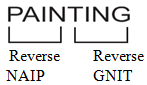Similarly,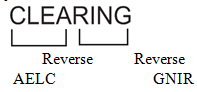Ashish > Prakash > Amir > Pramod

13 : 33 : : 2 : 53

Cloud cannot be formed from letter of given word.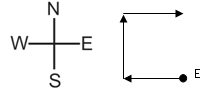Sun rises in East. That means he started walking towards West. Finally we will be facing east.

Directions: (Q. 10-12)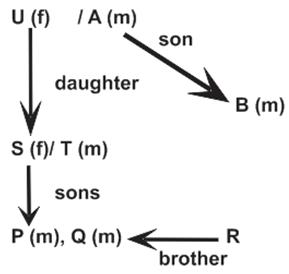6 = 2 × 3

24 = 6×  4

120 = 24 × 5

720 = 120 × 6

∴720 × 7 = 5040

16 square         – 1 × 1

9 square           – 2 × 2

4 square           – 3 × 3

1 square           – 4 × 4

Total = 30 square

D + 12   →   P

H + 12   →  T

L + 12   →   X

Similarly,

B + 12  →    N

F + 12   →   R

J + 12  →     V

### SSC Reasoning Ability Practice Questions – (Day-3)